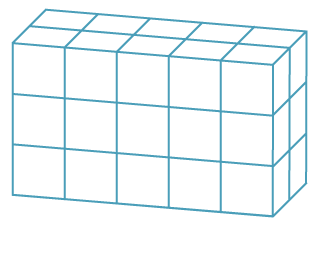### Associative law for multiplication

Multiplying three whole numbers corresponds geometrically to calculating the number of unit cubes in (or volume of) a rectangular prism.The volume is given by (5 × 2) × 3 = 5 × (2 × 3).

This is the associative law for multiplication.